Related Tags

loss function
regression losses

# What is a loss function?Sarvech Qadir

Loss functions are used to estimate and understand how well a particular algorithm performs. They are classified into two categories:

1. Regression Models are used to predict continuous values.

2. Classification Models are used to predict the particular output from a set of finite and categorical values.## Regression losses

### Mean Squared Error (MSE)

MSE is also referred to as quadratic or L2 loss. This method calculates the mean(average) of the square of the difference between predictions and actual observations.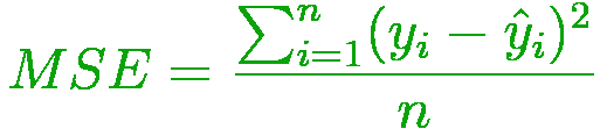### Mean Absolute Error (MAE)

MAE is also referred to as L1 loss. This method calculates the mean(average) of the sum of the absolute differences between predictions and actual observations.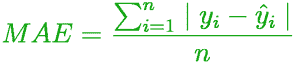### Mean Bias Error (MBE)

MBE is similar to MSE but less accurate. However, it is used to conclude if the model’s bias was negative or positive.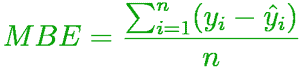### Huber Loss

Huber Loss is also referred to as Smooth Mean Absolute Error. This is an absolute error and often becomes a quadratic error when the error is too tiny. However, it is not that sensitive to outliers.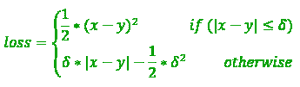## Classification losses

### Cross Entropy Loss

Cross Entropy Loss is also referred to as Negative Log Likelihood or Log Loss. It measures a classification model’s performance where the output is a probability value between 0 and 1. Cross-entropy loss increases as the predicted probability diverges from the actual label.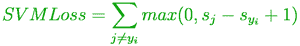### Hinge Loss

Hinge Loss is also referred to as a multi-class SVM loss. Hinge loss is applied for maximum-margin classification, most prominently for support vector machines (SVMs). It is used for training classifiers and is a convex function used in convex optimizers.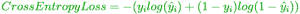RELATED TAGS

loss function
regression losses

CONTRIBUTORSarvech Qadir